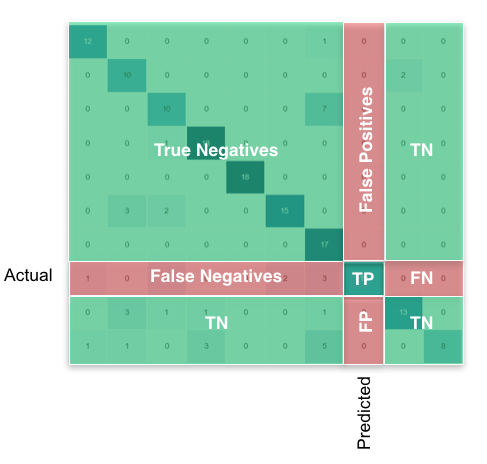A receiver operating characteristic curve, or ROC curve, is a graphical plot that illustrates the diagnostic ability of a binary classifier system as its discrimination threshold is varied. The method was originally developed for operators of military radar receivers#### Videos

Topic Access
Train, Test, & Validation Data Sets (7 min) https://www.youtube.com/watch?v=Zi-0rlM4RDs

ROC and AUC, Clearly Explained (16 min)
This is good  🙂

#### Articles

Medium Topic Access
Excercise Understanding Confusion Matrix in R https://www.datacamp.com/community/tutorials/confusion-matrix-calculation-r
Exercise with Answer Model Evaluation https://www.r-exercises.com/2016/12/02/model-evaluation-exercise-1/
Exercise with Answer Model Evaluation https://www.r-exercises.com/2016/12/22/model-evaluation-2/
Tutorial Lift Charts
Updated by IBM

https://www.ibm.com/docs/en/spss-statistics/28.0.0?topic=customers-cumulative-gains-lift-charts

Tutorial Generate ROC Curve Charts for Print and Interactive Use https://cran.r-project.org/web/packages/plotROC/vignettes/examples.html

# From a confusion matrix

condition positive (P)
the number of real positive cases
condition negative (N)
the number of real negative cases

true positive (TP)
True positive Prediction=A test result that correctly indicates the presence of a condition or characteristic
true negative (TN)
True negative Prediction=A test result that correctly indicates the absence of a condition or characteristic
false positive (FP)
False positive Prediction=A test result which wrongly indicates that a particular condition or attribute is present
false negative (FN)
False negative Prediction=A test result which wrongly indicates that a particular condition or attribute is absent

### sensitivity

sensitivityrecallhit rate, or true positive rate (TPR)

### specificity

specificityselectivity or true negative rate (TNR)### precision

precision or positive predictive value (PPV)
negative predictive value (NPV)miss rate or false negative rate (FNR)fall-out or false positive rate (FPR)false discovery rate (FDR)false omission rate (FOR)Positive likelihood ratio (LR+)Negative likelihood ratio (LR-)prevalence threshold (PT)threat score (TS) or critical success index (CSI)Prevalence### rpp     (Rate of Positive Predictions)

rpp = (tp+fp)/(tp+fn+fp+tn)

When rpp is 0.1, the number of times we have had some positive prediction are 10% of the total(regardless of our goodness)

### Accuracy

accuracy (ACC)balanced accuracy (BA)F1 score
is the harmonic mean of precision and sensitivityphi coefficient (φ or rφ) or Matthews correlation coefficient (MCC)Fowlkes–Mallows index (FM)informedness or bookmaker informedness (BM)markedness (MK) or deltaP (Δp)Diagnostic odds ratio (DOR)Sources: Fawcett (2006), Piryonesi and El-Diraby (2020), Powers (2011), Ting (2011), CAWCR, D. Chicco & G. Jurman (2020, 2021), Tharwat (2018).

==================================================================================

#To create a confusion matrix with complete analysis of measures

library(caret)

KNNconfusionMatrix<-caret::confusionMatrix(Predictionsvector,actualvector)
KNNconfusionMatrix

#Easiest and best visualization gain and lift in R:

#install.packages(‘CustomerScoringMetrics’)
library(CustomerScoringMetrics)
CustomerScoringMetrics::cumGainsChart(Predictionsvector,actualvector)
CustomerScoringMetrics::liftChart(Predictionsvector,actualvector)

# ROC gain lift With more elaboration in R

##################################################
#  Create a prediction object from predictions and actuals of any test data
# Then by Various performance analysis you will have ROC , cumulative gain and lift charts
# rpp = (tp+fp)/(tp+fn+fp+tn)      (Rate of Positive Predictions)
#################################################
ROCRpredictionObjfromAnyModel<-ROCR::prediction(as.numeric(PredictionsfromAnyTestset),as.numeric(ActualsfromAnyTestset))
plotableROC<- ROCR::performance(ROCRpredictionObjfromAnyModel,measure=”tpr”,x.measure=”fpr”)
plot(plotableROC, col=”orange”, lwd=2, main=”ROC curve for blah blah”)

plotableGain<- ROCR::performance(ROCRpredictionObjfromAnyModel,measure=”tpr”,x.measure=”rpp”)
plot(plotableGain, col=”orange”, lwd=2, main=”Gaincurve for blah blah”)
#################################################
# For example  we will  create a  prediction object from KNN model prediction vector
#####################################################

# Now we will use the prediction object we created above as a parameter that we pass to performance function
# this performance object will contain tpr and fpr
# ROC Chart:
ROCKNN <- ROCR::performance(ROCRpredictionObjfromKNN,measure=”tpr”,x.measure=”fpr”)

# if we plot tpr vs fpr it is ROC by definition 🙂
plot(ROCKNN, col=”orange”, lwd=2, main=”ROC curve ROCRpredictionObjKNN”)

# Now we will use the prediction object we created above as a parameter that we pass to performance function
# this performance object will contain tpr and rpp (Rate of Positive Predictions)

# if we plot tpr vs rpp it is Gains  chart by definition 🙂
# Gains Chart:
gainKNN <- ROCR::performance(ROCRpredictionObjfromKNN,measure=”tpr”,x.measure=”rpp”)
plot(gainKNN, col=”orange”, lwd=2, main=”gain curve KNN”)

# Lift Chart:
liftchartKNN<-ROCR::performance(ROCRpredictionObjfromKNN,”lift”,”rpp”)
plot(liftchartKNN, main=”Lift curve KNN”, colorize=T)

#AUC
library(ROCit)
roc_empirical <- ROCit::rocit(score = as.numeric(amirKNNextraxtedPredictionsvector), class = as.numeric(actualvectoradmitancefromtestdata), negref = 1)
# to get the area under the curve = AUC
summary(roc_empirical)
plot(roc_empirical)

=============================================

http://mlwiki.org/index.php/Cumulative_Gain_Chart

https://www.ncbi.nlm.nih.gov/pmc/articles/PMC4608333/

What is a gain chart?

https://idswater.com/2020/09/19/what-is-a-gain-chart/

http://www2.cs.uregina.ca/~dbd/cs831/notes/lift_chart/lift_chart.html

https://www.rdocumentation.org/packages/caret/versions/5.07-001/topics/predict.train

https://www.datanovia.com/en/lessons/determining-the-optimal-number-of-clusters-3-must-know-methods/

https://cran.r-project.org/web/packages/ROCit/index.html

=================================

https://en.wikipedia.org/wiki/Confusion_matrix

Other metrics can be included in a confusion matrix, each of them having their significance and use.

 Predicted condition Sources:  Total population = P + N Positive (PP) Negative (PN) Informedness, bookmaker informedness (BM) = TPR + TNR − 1 Prevalence threshold (PT) = TPR × FPR − FPR TPR − FPRActual condition Positive (P) True positive (TP), hit False negative (FN), type II error, miss, underestimation True positive rate (TPR), recall, sensitivity (SEN), probability of detection, hit rate, power = TP/P= 1 − FNR False negative rate (FNR), miss rate = FN/P= 1 − TPR Negative (N) False positive (FP), type I error, false alarm, overestimation True negative (TN), correct rejection False positive rate (FPR), probability of false alarm, fall-out = FP/N= 1 − TNR True negative rate (TNR), specificity (SPC), selectivity = TN/N= 1 − FPR Prevalence = P/P + N precision = TP/PP= 1 − FDR False omission rate (FOR) = FN/PN= 1 − NPV Positive likelihood ratio (LR+) = TPR/FPR Negative likelihood ratio (LR−) = FNR/TNR Accuracy (ACC) = TP + TN/P + N False discovery rate (FDR) = FP/PP= 1 − PPV Negative predictive value (NPV) = TN/PN= 1 − FOR Markedness (MK), deltaP (Δp) = PPV + NPV − 1 Diagnostic odds ratio (DOR) = LR+/LR− Balanced accuracy (BA) = TPR + TNR/2 F1 score = 2 PPV × TPR/PPV + TPR = 2 TP/2 TP + FP + FN Fowlkes–Mallows index (FM) = PPV × TPRMatthews correlation coefficient (MCC) = TPR × TNR × PPV × NPV− FNR × FPR × FOR × FDRThreat score (TS), critical success index (CSI), Jaccard index = TP/TP + FN + FP

## Confusion matrices with more than two categories

https://stackoverflow.com/questions/31324218/scikit-learn-how-to-obtain-true-positive-true-negative-false-positive-and-fal

Confusion matrix is not limited to binary classification and can be used in multi-class classifiers as well. The confusion matrices discussed above have only two conditions: positive and negative. For example, the table below summarizes communication of a whistled language between two speakers, zero values omitted for clarity.

Perceived
vowel
Vowel
produced
i e a o u
i 15   1
e 1   1
a     79 5
o     4 15 3
u       2 2Since 11 April 2023: 332 total views,  5 views today